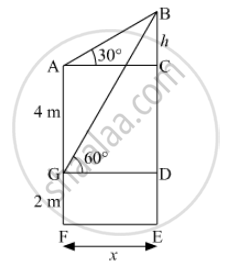Advertisement Remove all ads

# The Lower Window of a House is at a Height of 2 M Above the Ground and Its Upper Window is 4 M Vertically Above the Lower Window . at Certain Instant the Angles of Elevation of a Balloon Fr - Mathematics

Answer in Brief

The lower window of a house is at a height of 2 m above the ground and its upper window is 4 m vertically above the lower window . At certain instant the angles of elevation of a balloon from these windows are observed to be 600 and 30respectively. Find the height of the balloon above the ground.

Advertisement Remove all ads

#### SolutionLet the window G be 2 m above the ground.
Window A be 4 m above the window G.
Balloon be at point B above the ground.
In ∆ABC,

tan 30°= h/x

⇒ 1/sqrt3=h/x

⇒ x= h sqrt3       ................(1)

In ∆BGD,

tan 60°=(BD)/(GD)

⇒ sqrt3=( h+4)/x

⇒ x=( h+4)/sqrt3  ................(2)

From (i) and (ii) we get,

h sqrt3=( h+4)/sqrt3

⇒ 3h = h+4

⇒2h=4

⇒h=2

Hence, the height of the ballon above the ground = 2 + 4 + 2 = 8 m

Is there an error in this question or solution?
Advertisement Remove all ads

#### APPEARS IN

RD Sharma Class 10 Maths
Chapter 12 Trigonometry
Exercise 12.5 | Q 72 | Page 35
Advertisement Remove all ads

#### Video TutorialsVIEW ALL 

Advertisement Remove all ads
Share
Notifications

View all notifications

Forgot password?
Course